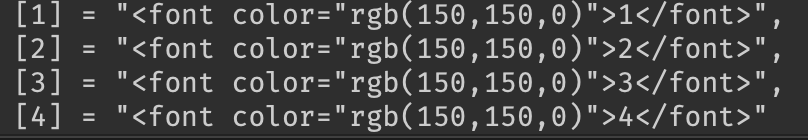# How do I replace all Integers in a string (RESOLVED)

I am making a Syntax Highlighting for my text but I wanna replace numbers.
When I do so it becomes
<font color="1<font color="1<font… (etc)
heres my code

``````NewData = NewData:gsub('%d', '<font color="rgb(150,150,0)">OKSO</font>')
``````

I dont know how to patch/fix this, I wanna make it so the “OKSO” string becomes the number I replaced with “%d”

``````data = '<font color="rgb(150,150,0)">OKSO</font>'
newData = data:gsub("OKSO", "%d") --replaces 'OKSO' with %d
``````

Nono I mean like I want this

``````



``````

to become

``````[<font color="rgb(150,150,0)">1</font>]
[<font color="rgb(150,150,0)">2</font>]
[<font color="rgb(150,150,0)">3</font>]
[<font color="rgb(150,150,0)">1</font>]
``````

When I do the gsub it replaces the numbers in rgb() aswell WHICH IS THE PROBLEM
(Also OKSO is an example)
(I want it for every number so 1234567890)

``````local tble = {1, 2, 3, 4}

for i, value in pairs(tble) do
tble[i] = '<font color="rgb(150,150,0)">'..i.."</font>"
end

``````

Already tried that, it did the same error as stated in the post. (it’s because I gsub “5” “0” and “1” which gsub rgb(150,150,0)

If i am understanding right, something like this:

``````local text = "number is 54321"
local newText = text:gsub("(%d+)", '<font color="rgb(150,150,0)">%1</font>') -- %1  refers to the captured digits
print(newText)
``````

Bit confused, it will not replace the numbers in RGB as they are different strings.

No, not how I am doing it. How I am doing it, it will edit them.

So, you want to replace any number inside two square brackets to a rich text formatted one without subtracting the brackets, right?

Here is an example implementation:

``````local NumberPattern = "%[(%d+)%]"
local Replacement = "[<font color=\"rgb(150,150,0)\">%1</font>]"

function Convert(Str: string)
return string.gsub(Str, NumberPattern, Replacement)
end

print(Convert("Some Text  another, "))  -- output: Some Text [<font color="rgb(150,150,0)">1</font>] another, [<font color="rgb(150,150,0)">2</font>]
``````

Just a little note, to make it support decimal, negative, and positive numbers you can modify the `NumberPattern` to `"%[([+-]?%d*%.?%d+)%]"` which will allow for that and will capture numbers like `-1.2`.

2 LikesI made my own demo in studio and I’m getting the results you want. If I’m not seeing something please point it out

``````local NewData = Data

NewData = NewData:gsub("(%d+)", '<font color="rgb(150,150,0)">%1</font>') -- %1  refers to the captured digits
NewData = NewData:gsub("local", '<font color="rgb(255,0,0)">local</font>')
NewData = NewData:gsub("function", '<font color="rgb(255,0,0)">local</font>')
NewData = NewData:gsub("self", '<font color="rgb(255,0,0)">local</font>')

return NewData``````

No, my script is different I’m using a loop to go through the entire table.

``````local tble = {1, 2, 3, 4}

for i, value in pairs(tble) do
tble[i] = '<font color="rgb(150,150,0)">'..i.."</font>"
end

``````

IM LAGGING EOIHEKBRG
but this worked ty I needed to know how to edit the certain digit and replaceHow my script works it can’t use that.

What do you mean? Can you explain more?

This topic was automatically closed 14 days after the last reply. New replies are no longer allowed.